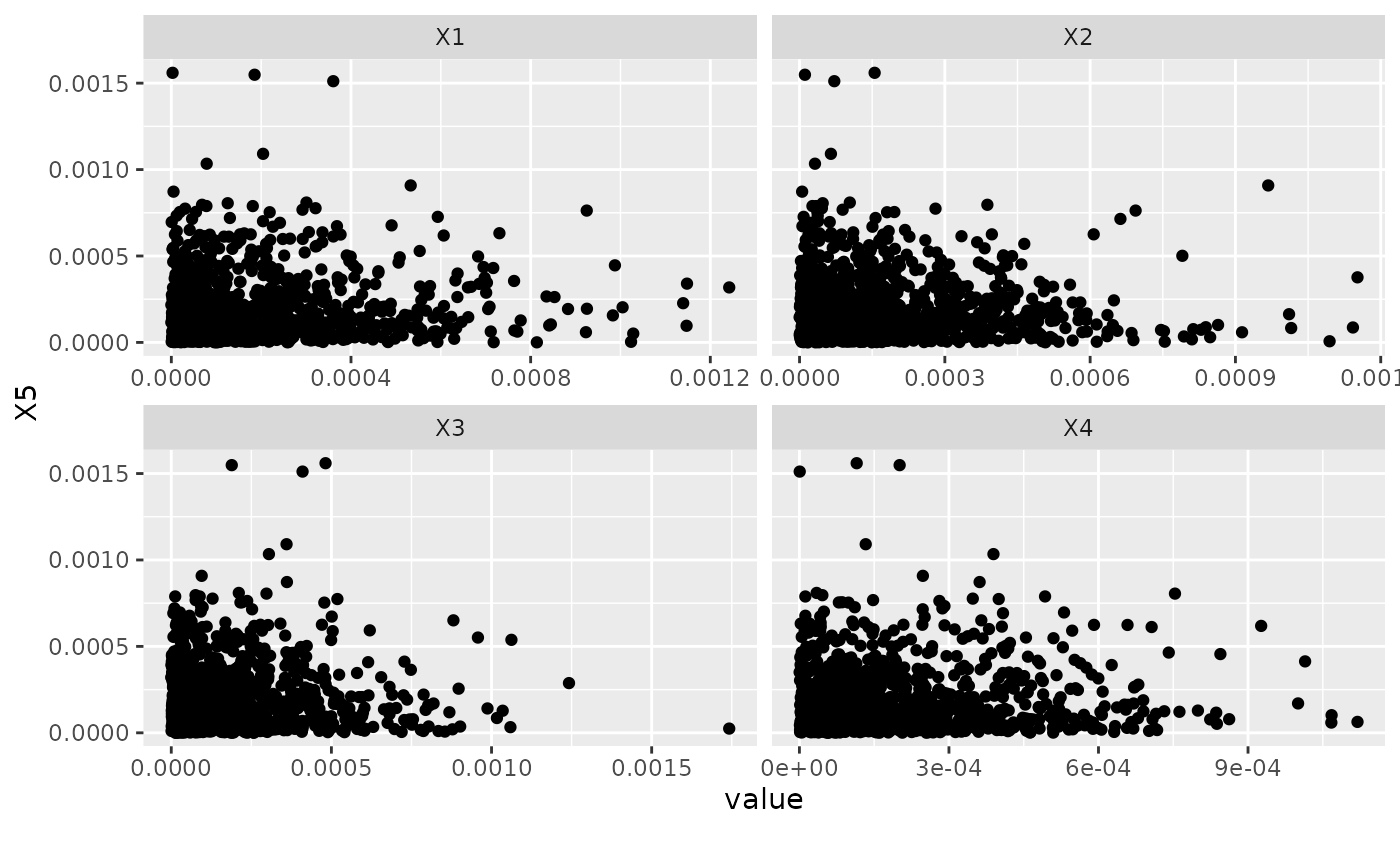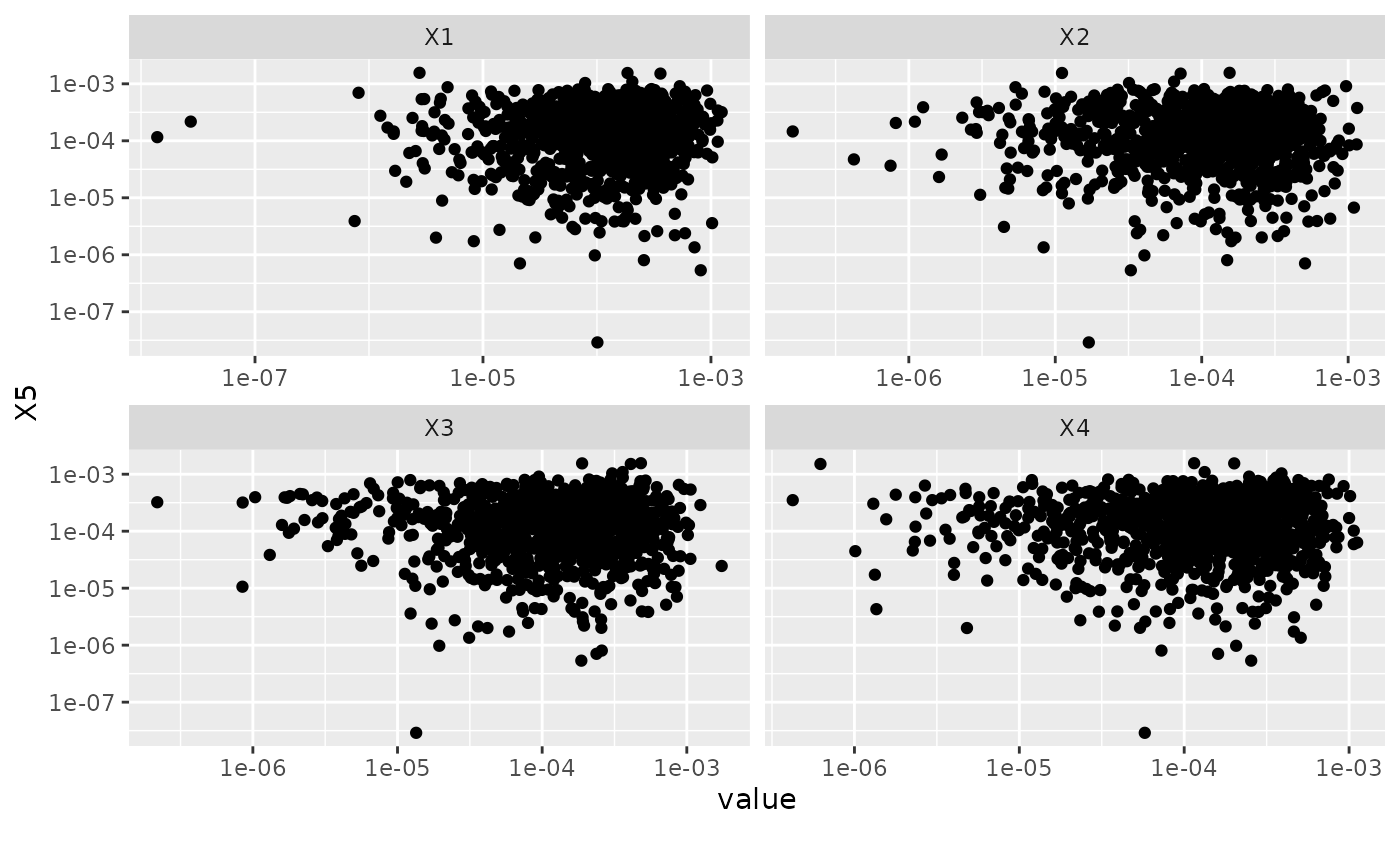This function creates scatterplot for all features fixing on a selected feature.

plot_scatterplot(
data,
by,
sampled_rows = nrow(data),
geom_point_args = list(),
scale_x = NULL,
scale_y = NULL,
title = NULL,
ggtheme = theme_gray(),
theme_config = list(),
nrow = 3L,
ncol = 3L,
parallel = FALSE
)

## Arguments

data input data feature name to be fixed at number of rows to sample if data has too many rows. Default is all rows, which means do not sample. a list of other arguments to geom_point scale of original x axis (before coord_flip). See scale_x_continuous for all options. Default is NULL. scale of original y axis (before coord_flip). See scale_y_continuous for all options. Default is NULL. plot title complete ggplot2 themes. The default is theme_gray. a list of configurations to be passed to theme. number of rows per page number of columns per page enable parallel? Default is FALSE.

## Value

invisibly return the named list of ggplot objects

## Examples

plot_scatterplot(iris, by = "Species")# Plot skewed data on log scale
set.seed(1)
skew <- data.frame(replicate(5L, rbeta(1000, 1, 5000)))
plot_scatterplot(skew, by = "X5", ncol = 2L)plot_scatterplot(skew, by = "X5", scale_x = "log10", scale_y = "log10", ncol = 2L)if (FALSE) {
# Customize themes
library(ggplot2)
plot_scatterplot(
data = mpg,
by = "hwy",
geom_point_args = list(size = 1L),
theme_config = list("axis.text.x" = element_text(angle = 90)),
ncol = 4L
)
}